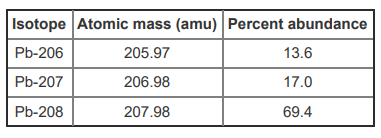# Problem: In the PhET simulation, click on the Mixtures tab. Select Mg from the periodic table. Drag the colored spheres representing the different isotopes of magnesium into the black background. Notice how the amount of any particular isotope in comparison to the others affects the percent composition and the average atomic mass. In the bottom right-hand corner, click on Nature's Mix to see how the selected element's isotopes appear in nature.Part D.Suppose the given data represent the abundance of isotopes of an element. Calculate the average atomic mass of lead based on the given data and the atomic masses.Express your answer to four significant figures.Percent abundance and average atomic mass The atomic mass of an element is calculated based on the atomic masses of each of its naturally occurring isotopes as follows: atomic mass = [(mass of isotope 1)(abundance of isotope 1)                                    + (mass of isotope 2)(abundance of isotope 2) + ⋯ ] The isotopes with a higher abundance receive more weight in the equation and therefore contribute more to the value of the average atomic mass

###### FREE Expert SolutionView Complete Written Solution
###### Problem Details

In the PhET simulation, click on the Mixtures tab. Select Mg from the periodic table. Drag the colored spheres representing the different isotopes of magnesium into the black background. Notice how the amount of any particular isotope in comparison to the others affects the percent composition and the average atomic mass. In the bottom right-hand corner, click on Nature's Mix to see how the selected element's isotopes appear in nature.

Part D.Suppose the given data represent the abundance of isotopes of an element. Calculate the average atomic mass of lead based on the given data and the atomic masses.Percent abundance and average atomic mass The atomic mass of an element is calculated based on the atomic masses of each of its naturally occurring isotopes as follows:

The isotopes with a higher abundance receive more weight in the equation and therefore contribute more to the value of the average atomic mass